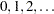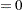CONTRAST Statement
CONTRAST ’label’ values ;

This statement is used to identify tests between the levels of the CLASS variable; in particular, it is used to specify the coefficients for the trend tests. The label is a string naming the contrast; it contains a maximum of 21 characters. The values are scoring coefficients across the CLASS variable levels.

You can specify multiple CONTRAST statements, thereby specifying multiple contrasts for each variable. Multiplicity adjustments are computed for all contrasts and all variables simultaneously. The coefficients are applied to the ordered CLASS variables; this order can be changed with the ORDER= option in the PROC MULTTEST statement. For example, consider a four-group experiment with CLASS variable levels A1, A2, B1, and B2 denoting two levels of two treatments. The following statements produce three linear trend tests for each variable identified in the TEST statement. PROC MULTTEST computes the multiplicity adjustments over the entire collection of tests, which is three times the number of variables.

```contrast 'a vs b'    -1 -1  1  1;
contrast 'a linear'  -1  1  0  0;
contrast 'b linear'   0  0 -1  1;
```

As another example, consider an animal carcinogenicity experiment with dose levels 0, 4, 8, 16, and 50. You can specify a trend test with the indicated scoring coefficients by using the following statement:

```contrast 'arithmetic trend' 0 4 8 16 50;
```

Multiplicity-adjusted p-values are then computed over the collection of variables identified in the TEST statement. See Lagakos and Louis (1985) for guidelines on the selection of contrast-scoring values.

When a Fisher test is specified in the TEST statement, the CONTRAST statement coefficients are used to group the CLASS variable’s levels. Groups with acontrast coefficient are combined and compared with groups with a 1 contrast coefficient for each test, and groups with a 0 coefficient are not included in the contrast. For example, the following statements compute Fisher exact tests for (a) control versus the combined treatment groups, (b) control versus the first treatment group, and (c) control versus the third treatment group:

```contrast 'c vs all' 1 -1 -1 -1;
contrast 'c vs t1'  1 -1  0  0;
contrast 'c vs t3'  1  0  0 -1;
```

Multiplicity adjustments are then computed over the entire collection of tests and variables. Only,, andare acceptable CONTRAST coefficients when the Fisher test is specified; PROC MULTTEST ignores the CONTRAST statement if any other coefficients appear.

If you specify the FISHER test and no CONTRAST statements, then all contrasts of control versus treatment are automatically generated, with the first level of the CLASS variable deemed to be the control. In this case, the control level is assigned the valuein each contrast and the other treatment levels are assigned. You should therefore use the LOWERTAILED option to test for higher success rates in the treatment groups.

For tests other than FISHER, CONTRAST values areby default. If you specify the CA or PETO test with the PERMUTATION= option, then your CONTRAST coefficients must be integer valued.

For t tests for the mean of continuous data (and for the FT tests), the contrast coefficients are centered to have mean. The resulting centered scoring coefficients are then applied to the sample means (or to the double-arcsine-transformed proportions in the case of the FT tests).